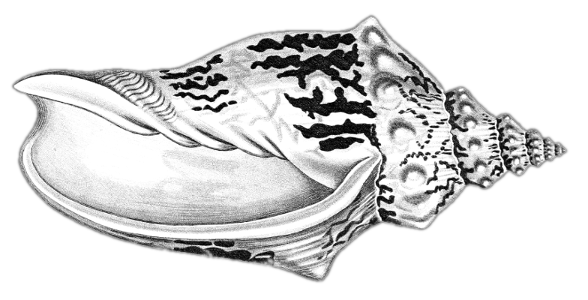In this lesson module, you'll learn the mechanics of a padding oracle attack. Much more detail about the context of this attack is given in chapter 10 of the textbook.

# CBC Malleability​

Here's what CBC mode decryption looks like (with 16-bit blocks):
ciphertext = F-1 ⊕
What happens when you flip some bits in the first ciphertext block? What is the effect on the resulting plaintext? Try it! (click on ciphertext bits to flip them)

Let's consider a padding scheme where correctly padded blocks end in either

0001
or
0000
0010
or
0000
0000
0011
or
0000
0000
0000
0100

See if you can flip some bits in this ciphertext so that it decrypts to something with valid padding. Try it:

ciphertext = valid padding? no F-1 ⊕

Look at the bits you had to flip to achieve valid padding (the red bits). What is the relationship between those bits and the bits of the original plaintext?

# Attack​

Now suppose the only information you can see is whether flipping certain ciphertext bits results in a plaintext with valid padding. Can you use this ability to decrypt the ciphertext? Try it!

When your guess of the plaintext is correct, it will turn green. If you need to "cheat," you can look at the entire result of decryption.

ciphertext = (show/hide decryption) F-1 ⊕ no

Suggestions:

• Try to find a way to flip the last 4 bits that results in valid padding.
• Now you know that the (modified) plaintext must end in
0001
, so you should be able to figure out what the original plaintext was.
• Flip more bits to try to get the (modified) plaintext to end in
0000
0010
.
• etc etc